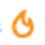# i=1?

We know that $i=\sqrt { -1 }$

But $i={ i }^{ 4\times \frac { 1 }{ 4 } }\\ { ({ i }^{ 4 }) }^{ \frac { 1 }{ 4 } }\\ { 1 }^{ \frac { 1 }{ 4 } }\\ =1$

So does that mean i=1? Please helpNote by Satyajit Ghosh
5 years, 5 months ago

This discussion board is a place to discuss our Daily Challenges and the math and science related to those challenges. Explanations are more than just a solution — they should explain the steps and thinking strategies that you used to obtain the solution. Comments should further the discussion of math and science.

When posting on Brilliant:

• Use the emojis to react to an explanation, whether you're congratulating a job well done , or just really confused .
• Ask specific questions about the challenge or the steps in somebody's explanation. Well-posed questions can add a lot to the discussion, but posting "I don't understand!" doesn't help anyone.
• Try to contribute something new to the discussion, whether it is an extension, generalization or other idea related to the challenge.
• Stay on topic — we're all here to learn more about math and science, not to hear about your favorite get-rich-quick scheme or current world events.

MarkdownAppears as
*italics* or _italics_ italics
**bold** or __bold__ bold
- bulleted- list
• bulleted
• list
1. numbered2. list
1. numbered
2. list
Note: you must add a full line of space before and after lists for them to show up correctly
paragraph 1paragraph 2

paragraph 1

paragraph 2

[example link](https://brilliant.org)example link
> This is a quote
This is a quote
    # I indented these lines
# 4 spaces, and now they show
# up as a code block.

print "hello world"
# I indented these lines
# 4 spaces, and now they show
# up as a code block.

print "hello world"
MathAppears as
Remember to wrap math in $$ ... $$ or $ ... $ to ensure proper formatting.
2 \times 3 $2 \times 3$
2^{34} $2^{34}$
a_{i-1} $a_{i-1}$
\frac{2}{3} $\frac{2}{3}$
\sqrt{2} $\sqrt{2}$
\sum_{i=1}^3 $\sum_{i=1}^3$
\sin \theta $\sin \theta$
\boxed{123} $\boxed{123}$

## Comments

Sort by:

Top Newest

Hmm. Good. What if we write it as $(1^ \frac {1}{2} )^ \frac{1}{2}$ , then square root of 1 is -1. Then you will get the desired result. Your working is a result of false root. :-)

- 5 years, 5 months ago

Log in to reply

It's what Margaret was talking about but even if there is a false root this means 1 is a root of i irrespective of it being false or true.

- 5 years, 5 months ago

Log in to reply

Yup. That is root. (Although that is false)

- 5 years, 5 months ago

Log in to reply

Here is what I think: 1^0.25=1/1^4. just as x^2=k(k is any constant) has 2 solutions (whether it is within the range of real number or not), x^4=k should have 4 solutions. Think that you are solving an equation 1/x^4 = 1. i and 1 are both solutions (two out of the four) of the equation 1/x^4=1.

- 5 years, 5 months ago

Log in to reply

I totally agree that it has 4 solutions, but if we open x then it will have 1 as a solution of i. Although there maybe 3 more but 1 is also its solution right?

- 5 years, 5 months ago

Log in to reply

i can also be written as i^n×1/n and then i will have n roots. So now can we say that i has n values?

- 5 years, 5 months ago

Log in to reply

×

Problem Loading...

Note Loading...

Set Loading...